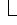# Range Object

DataSheetRange

Represents a cell, a row, a column, or a selection of cells that contains one or more contiguous blocks of cells.

## Using the Range Object

The following properties for returning a Range object are described in this section:

• Range property
• Cells property

## Range Property

Use Range(arg), where arg is the name of the range, to return a Range object that represents a single cell or a range of cells. The following example places the value of cell A1 in cell A5.

``````myChart.Application.DataSheet.Range("A5").Value = _
myChart.Application.DataSheet.Range("A1").Value``````

The following example fills the range A1:H8 with the value 20.

``myChart.Application.DataSheet.Range("A1:H8").Value = 20``

## Cells Property

Use Cells(row, column), where row is the row's index number and column is the column's index number, to return a single cell. The following example sets the value of cell A1 to 24 (column A is the second column on the datasheet, and row 1 is the second row on the datasheet).

``myChart.Application.DataSheet.Cells(2, 2).Value = 24``

Although you can also use `Range("A1")` to return cell A1, there may be times when the Cells property is more convenient because you can use a variable for the row or column. The following example creates column and row headings on the datasheet.

``````Sub SetUpTable()
With myChart.Application.DataSheet
For theYear = 1 To 5
.Cells(1, theYear + 1).Value = 1990 + theYear
Next theYear
For theQuarter = 1 To 4
.Cells(theQuarter + 1, 1).Value = "Q" & theQuarter
Next theQuarter
End With
End Sub``````

Although you can use Visual Basic string functions to alter A1-style references, it's much easier (and much better programming practice) to use the `Cells(1, 1)` notation.

Use expression.Cells(row, column), where expression is an expression that returns a Range object, and row and column are relative to the upper-left corner of the range, to return part of a range. The following example sets the value for cell C5.

``myChart.Application.Range("C5:C10").Cells(1, 1).Value = 35``